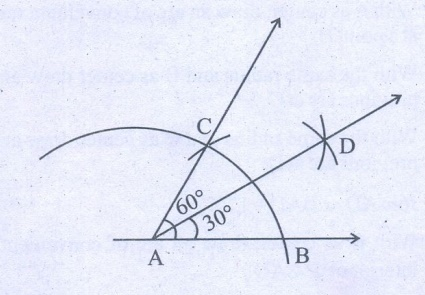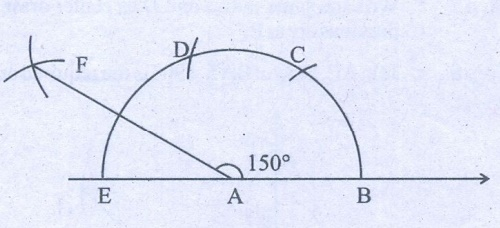Home | | Maths 7th Std | Exercise 5.5 (Construction of special angles without using protractor)

# Exercise 5.5 (Construction of special angles without using protractor)

7th Maths : Term 1 Unit 5 : Geometry : Construction of special angles without using protractor

Exercise 5.5

1. Construct the following angles using ruler and compass only.

(i) 60°

(ii) 120°

(iii) 30°

(iv) 90°

(v) 45°

(vi) 150°

(vii) 135

(i) 60oStep 1 : Draw a line. Mark a point A on it.

Step 2 : With A as center draw an arc of convenient radius, to the line at a point B.

Step 3 : With the same radius and B as center draw an arc to cut the previous arc at C.

Step 4 : Join AC. Now BAC is the required angle with the measure 60°.

(ii) 120°Step 1 : Draw a line. Mark a point A on it.

Step 2 : With A as center, draw an arc of convenient radius to the line at a point B.

Step 3 : With the same radius and B as center draw an arc to cut the previous arc at C.

Step 4 : With the same radius and C as center draw an arc to cut previous arc at D.

Step 5 : Join AD. Now BAD is the required angle with the measure 120°.

(iii) 30°Step 1 : Draw a line. Mark a point A on it.

Step 2 : With A as center, draw an arc of convenient radius to the line at a point B.

Step 3 : With the same radius and B as center draw an arc to cut the previous arc at C.

Step 4 : Join AC. BAC = 60°

Step 5 : With B as center, draw an arc of convenient radius in the interior of BAC.

Step 6 : With the same radius and C as center draw an arc to cut the previous arc at D.

Step 7 : Join AD. Now BAD is the required angle with measure 30°.

(iv) 90oStep 1 : Draw a line. Mark a point A on it.

Step 2 : With A as center, draw an arc of convenient radius to the line at a point B.

Step 3 : With the same radius and B as center draw an arc to cut the previous arc at C.

Step 4 : With the same radius and C as center draw an arc to cut the previous arc at D.

Step 6 : With C as center, draw an arc of convenient radius in the interior of CAD

Step 7 : With the same radius and D as center draw an arc to cut the previous arc at E.

Step 8 : Join AE. Now BAE = 90° is the required angle.

(v) 45oStep 1 : Draw a line. Mark a point A on it.

Step 2 : With A as center, draw an arc of convenient radius to the line at a point B.

Step 3 : With the same radius and B as center draw an arc to cut the previous arc at C.

Step 4 : With the same radius and C as center draw an arc to cut the previous arc at D.

Step 6 : With C as center, draw an arc of convenient radius in the interior of CAD.

Step 7 : With the same radius and D as center draw an arc to cut the previous arc at E.

Step 8 : Join AE. It cuts the previous BCD arc at F B AF = 90°.

Step 9 : With B and F as centers draw arcs of convenient radius in the interior of BAF. They cut G.

Step 10 : Join AG Now BAG the required angle with measure 45°.

(vi) 150°Step 1 : Draw a line. Mark a point A on it.

Step 2 : With A as center, draw an arc of convenient radius to the line at a point B.

Step 3 : With the same radius and B as center draw an arc to cut the previous arc at C.

Step 4 : With the same radius and C as center draw an arc to cut the previous arc at D.

Step 5 : With the same radius and D as center draw an arc to cut the previous arc at E.

Step 6 : With D and E as centers and with the convenient radius draw arcs in the interior of DAE to cut at F.

Step 7 : JoinAF. Now BAF = 150° is the required angle.

(vii) 135oStep 1 : Draw a line. Mark a point A on it.

Step 2 : With A as center, draw an arc of convenient radius to the line at a point B.

Step 3 : With the same radius and B as center draw an arc to cut the previous arc at C.

Step 4 : With the same radius and C as center draw an arc to cut the previous arc at D.

Step 5 : With the same radius and D as center draw an arc to cut the previous arc at E.

Step 6 : With the convenient radius and D and E as centers draw arcs in the interior of DAE to cut at F.

Step 7 : Join AF. It cuts the arc at G. Now GAB = 150°

Step 8 : With the convenient radius and G and D as centers draw arcs in the interior of DAG to cut at H.

Step 9 : Join AH. Now BAH = 135° is the required angle.

Tags : Questions with Answers, Solution | Geometry | Term 1 Chapter 5 | 7th Maths , 7th Maths : Term 1 Unit 5 : Geometry
Study Material, Lecturing Notes, Assignment, Reference, Wiki description explanation, brief detail
7th Maths : Term 1 Unit 5 : Geometry : Exercise 5.5 (Construction of special angles without using protractor) | Questions with Answers, Solution | Geometry | Term 1 Chapter 5 | 7th Maths